# rasterVis

## Introduction

The `rasterVis` package complements the `raster` and the `terra` packages, providing a set of methods for enhanced visualization and interaction. It defines visualization methods for quantitative data and categorical data, with `levelplot`, both for univariate and multivariate rasters.

It also includes several methods in the frame of the Exploratory Data Analysis approach: scatterplots with `xyplot`, histograms and density plots with `histogram` and `densityplot`, violin and boxplots with `bwplot`, and a matrix of scatterplots with `splom`.

On the other hand, `rasterVis` provides three methods to display spatiotemporal rasters: `hovmoller` produces Hovmöller diagrams, `horizonplot` creates horizon graphs, with many time series displayed in parallel, and `xyplot` displays conventional time series plots extracted from a multilayer raster.

Finally, this package is able to display vector fields using arrows, `vectorplot`, or with streamlines, `streamplot`.

This webpage illustrates some functionalities with examples. If you need more information, you may be interested in my book “Displaying Time Series, Spatial, and Space-Time Data with R”. It includes four chapters devoted to the visualization of spatial and spatiotemporal raster data. Along with the main graphics from the text, its website offers access to the datasets used in the examples as well as the full R code.

### Installation

The stable release of `rasterVis` can be found at CRAN. The development version is at GitHub.

Install the stable version with:

```install.packages('rasterVis')
```

You can install the development version with the remotes package:

```remotes::install_github('oscarperpinan/rasterVis')
```

or with the devtools package:

```devtools::install_github('oscarperpinan/rasterVis')
```

## Level plots

This section discusses how to display quantitative data with `levelplot` with an example using data from the CM SAF project, as described here.

```library(raster)
library(rasterVis)

old <- setwd(tempdir())
'SISmm2008_CMSAF.zip', method='wget')
unzip('SISmm2008_CMSAF.zip')

listFich <- dir(pattern='\\.nc')
stackSIS <- stack(listFich)

idx <- seq(as.Date('2008-01-15'), as.Date('2008-12-15'), 'month')

SISmm <- setZ(stackSIS, idx)
names(SISmm) <- month.abb

setwd(old)
```

Once the `Rasterstack` has been defined, it can be displayed easily with `levelplot`. Each panel of the graphic shows a layer of the `RasterStack` object using a trellis chart or small-multiple technique.

```levelplot(SISmm)
```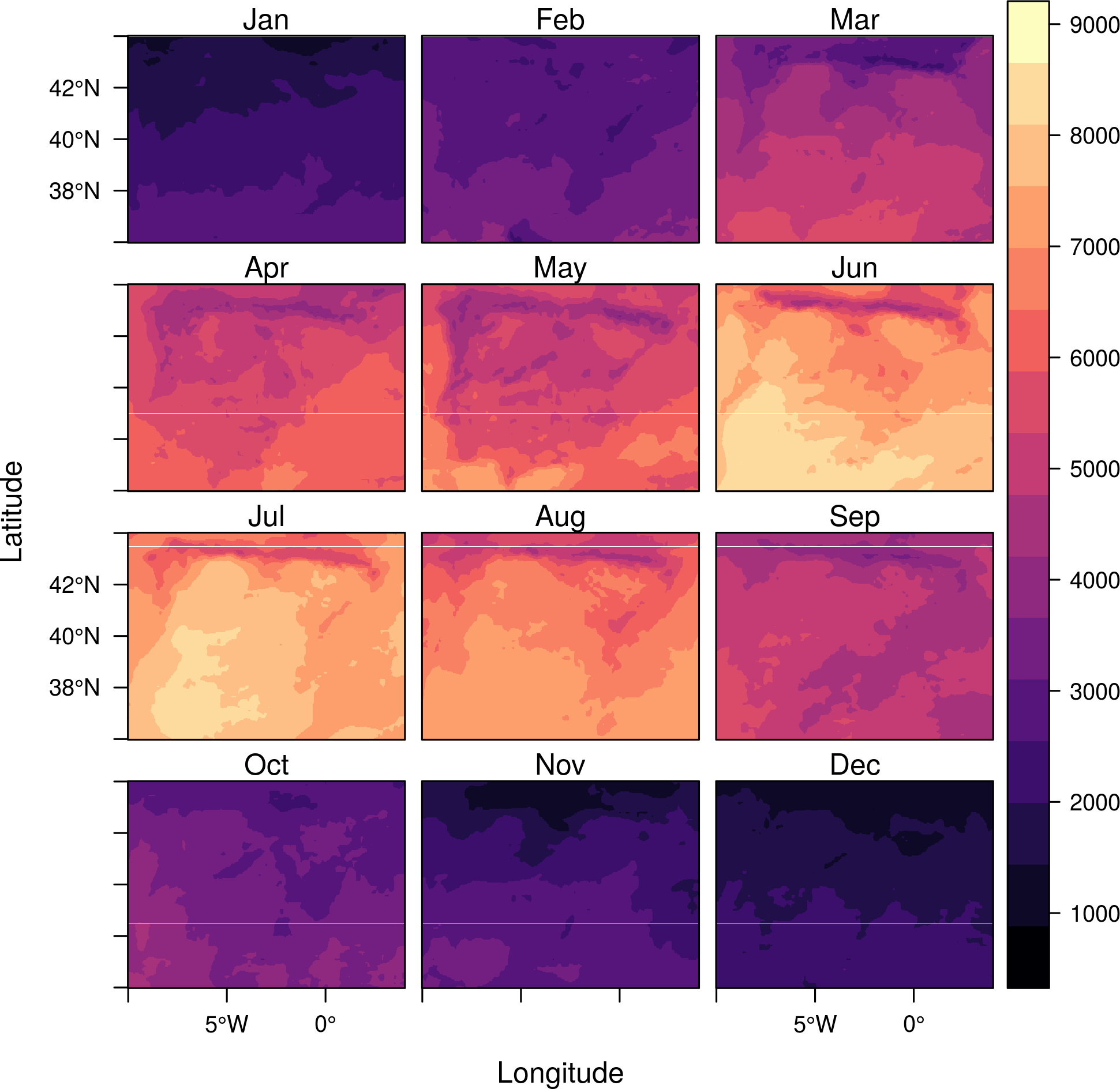If only one layer is chosen, this method displays two marginal plots, the row and column summaries of the `RasterLayer`, computed with the function defined by the component `FUN` of the list `margin` (which uses `mean` as default value):

```levelplot(SISmm, layers = 1, margin = list(FUN = 'median'), contour=TRUE)
```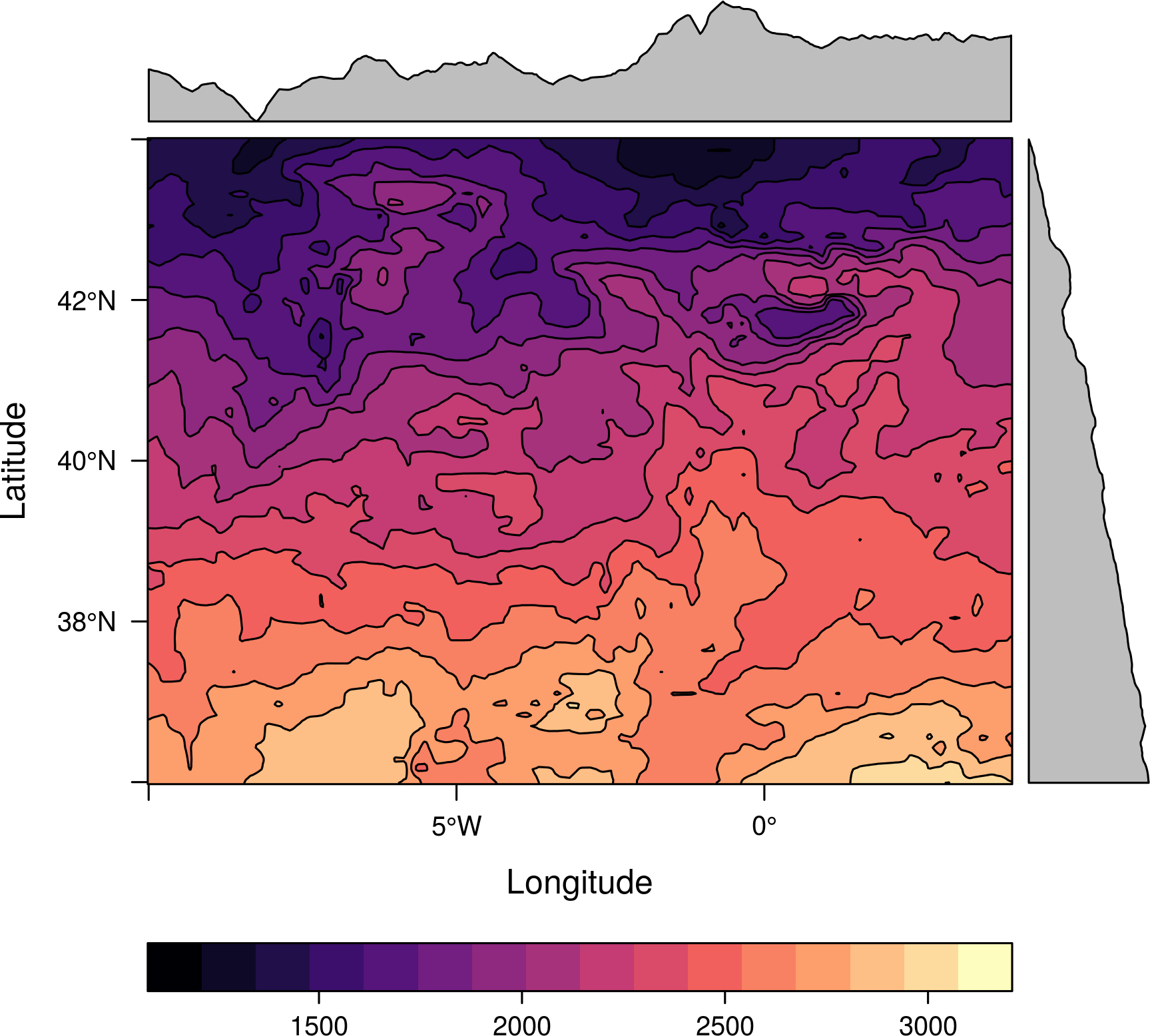### Overlay plots

The result of the last call is a `trellis` object. The latticeExtra package provides the `layer` function to add contents. For example, let’s add the administrative borders. This information is available at the GADM service.

```library(maptools)
proj <- CRS('+proj=longlat +ellps=WGS84')
##Modify next line to your folder

p <- levelplot(SISmm, layers=1, margin = list(FUN = median))
p + layer(sp.lines(mapaSHP, lwd=0.8, col='darkgray'))
```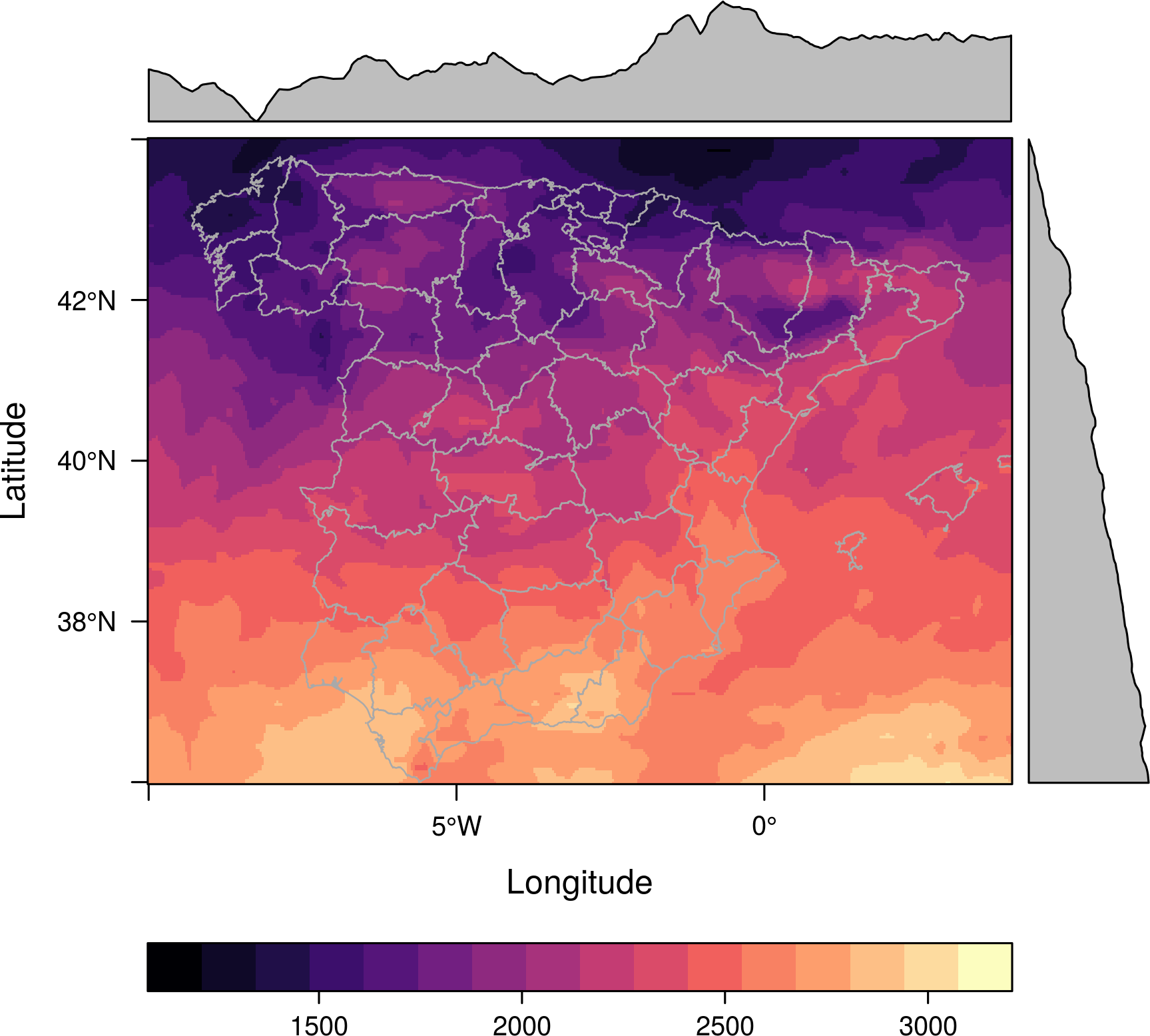A similar approach can be used to overlay several level plots. The solution uses the `+.trellis` mechanism implemented in `latticeExtra`. Let’s create two different `RasterLayer` objects:

```f <- system.file("external/test.grd", package="raster")
r <- raster(f)
r0 <- init(r, fun = rnorm)
```

… and create two levelplots with different color palettes:

```p0 <- levelplot(r0, par.settings = GrTheme)
p1 <- levelplot(r, par.settings = magmaTheme)
```

These plots can be easily combined using `+` (the first plot sets the color scale):

```p0 + p1
```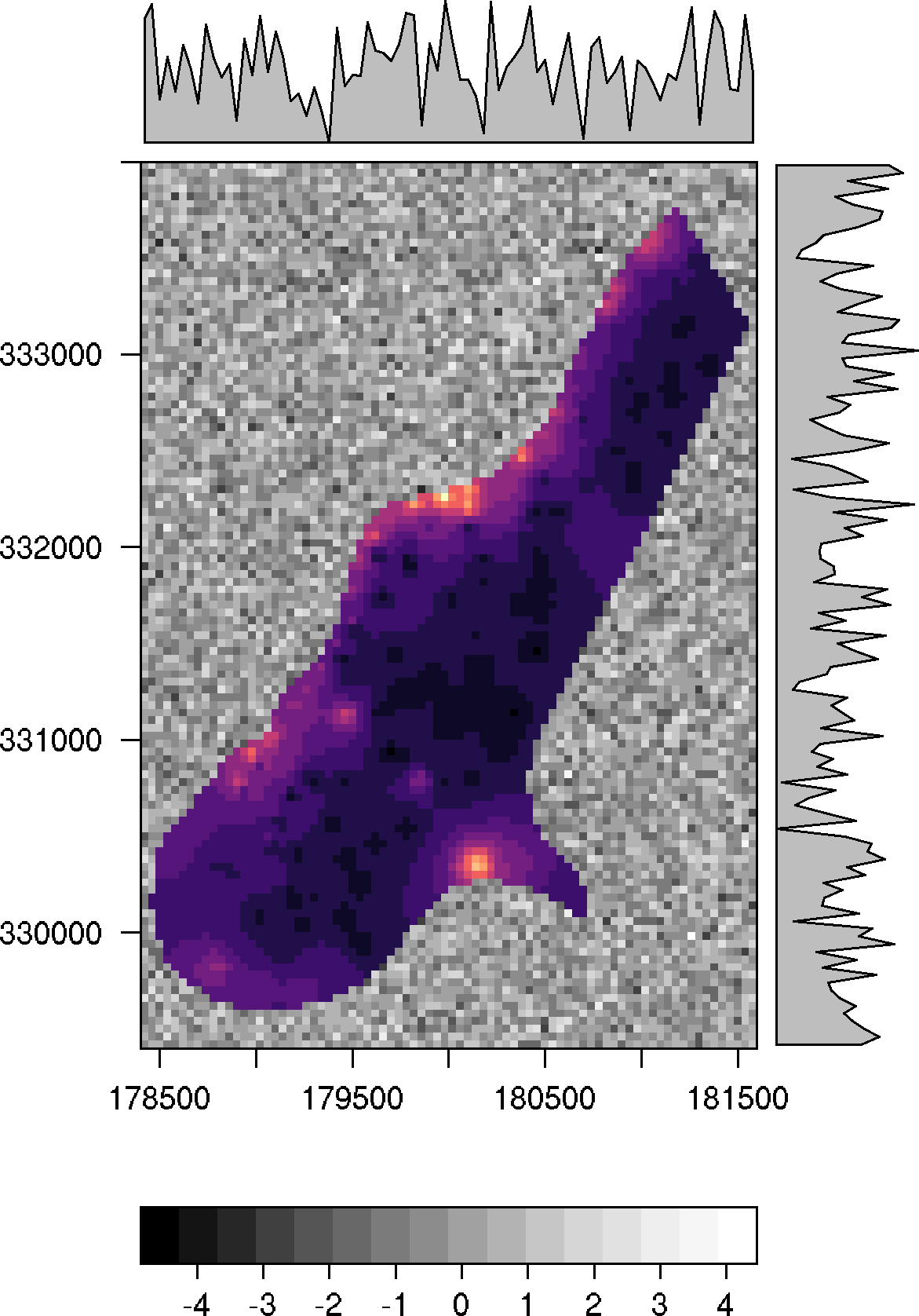The function `as.layer` with `under = TRUE` must be used in order to show the other color scale:

```p1 + as.layer(p0, under = TRUE)
```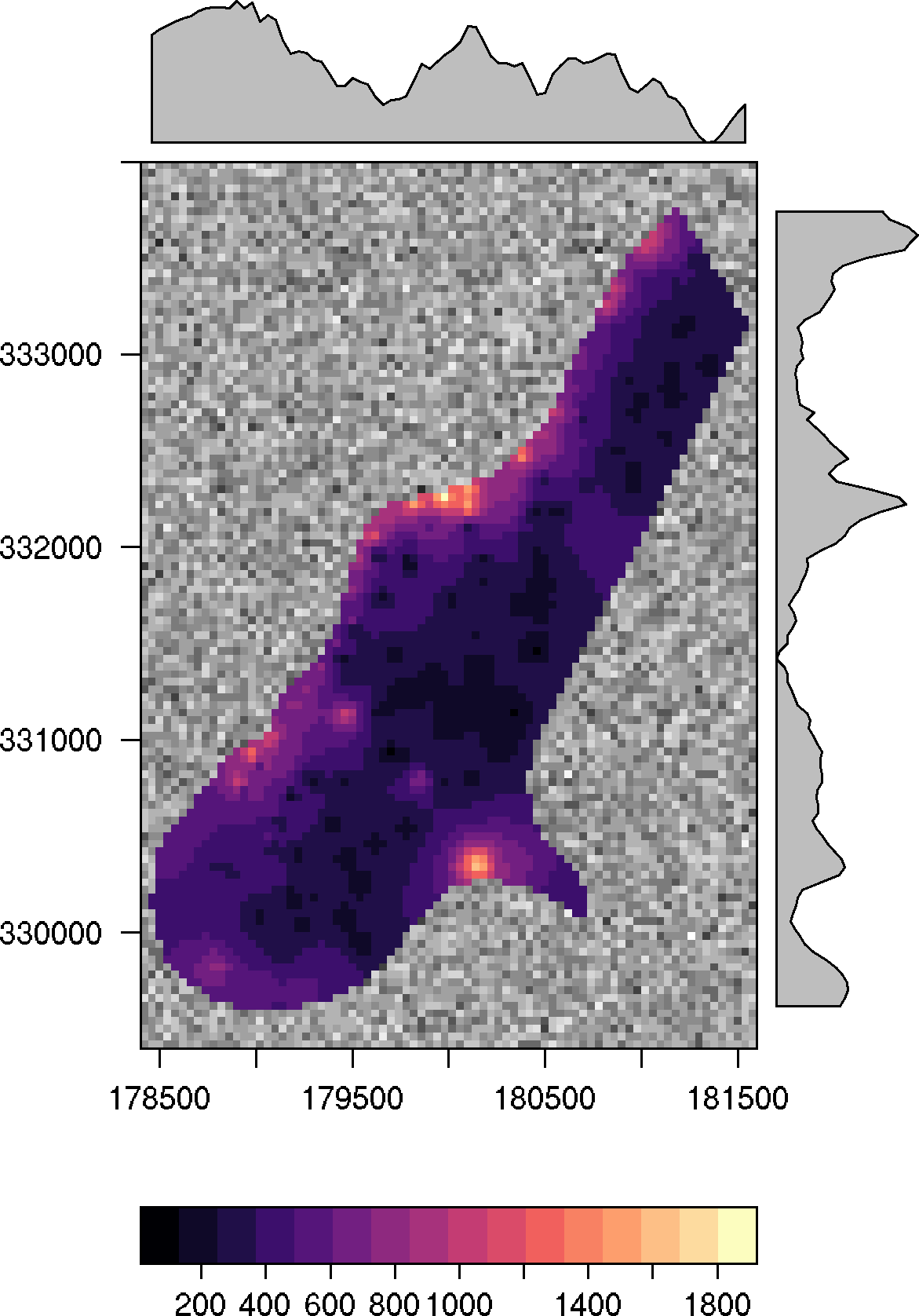### Log scale

The `zscaleLog` argument controls whether the object will be log transformed before being passed to the panel function. Defaults to ‘NULL’, in which case the Raster* is not transformed. Other possible values are any number that works as a base for taking logarithm, ‘TRUE’ (which is equivalent to 10), and ‘“e”’ (for the natural logarithm). As a side effect, the colorkey is labeled differently.

```f <- system.file("external/test.grd", package="raster")
r <- raster(f)
levelplot(r^2, zscaleLog=TRUE, contour=TRUE)
```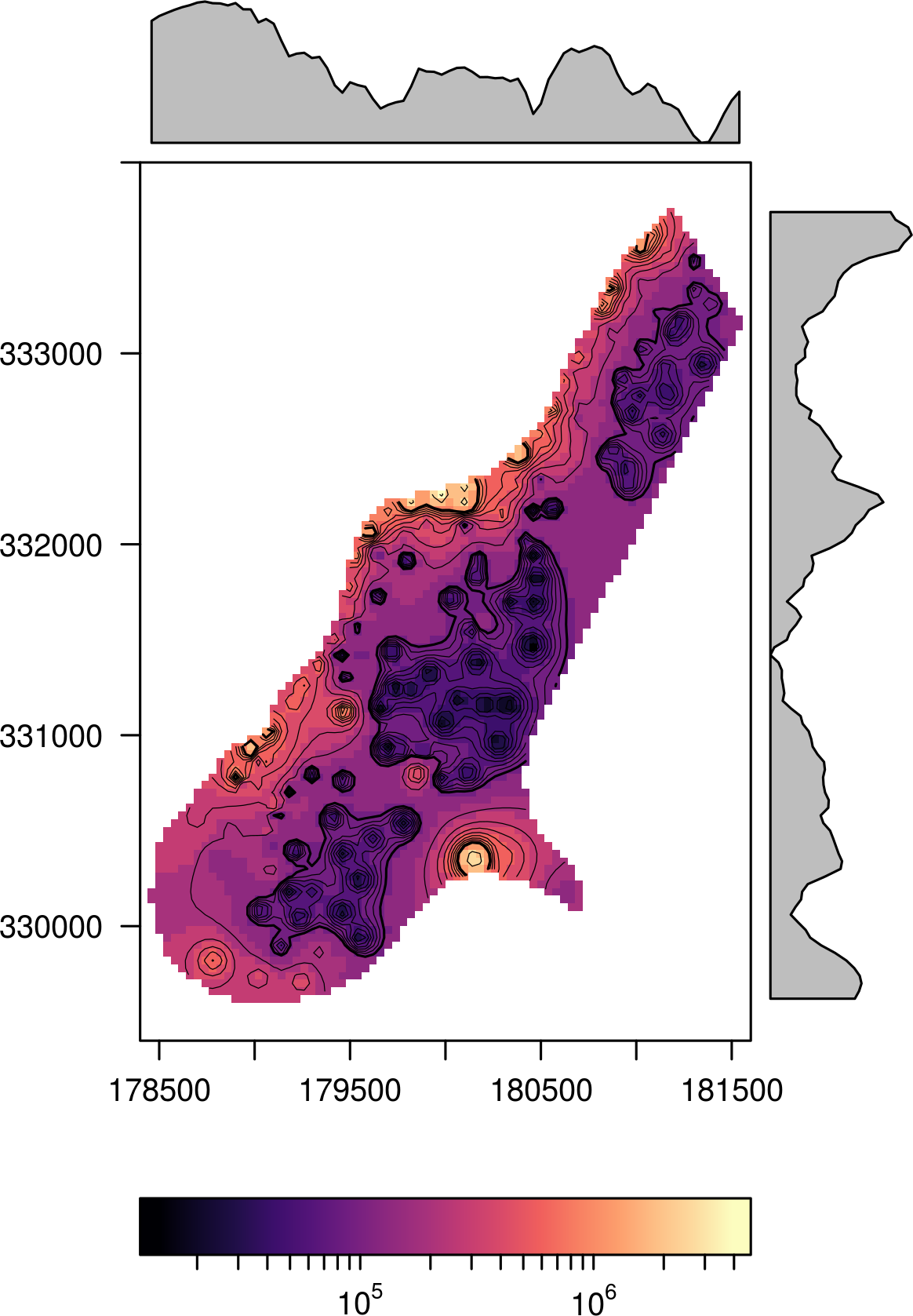## Themes

The previous plots used the default theme of rasterVis, `rasterTheme`, using the `magma` palette provided by the `viridisLite` package. The other palettes provided by this package are available through the `viridisTheme`, `infernoTheme`, and `plasmaTheme` functions. Besides, `YlOrRdTheme`, `BuRdTheme`, `RdBuTheme`, `GrTheme`, and `BTCTheme` are variations of `rasterTheme` using palettes of the `RColorBrewer` and `hexbin` packages. Let’s try them with an example:

```Aug <- raster(SISmm, 8)
```

and its overall mean is calculated with cellStats:

```meanAug <- cellStats(Aug, mean)
```

The `RdBuTheme` diverging palette is specially well suited to this data:

```levelplot(Aug - meanAug, par.settings = RdBuTheme)
```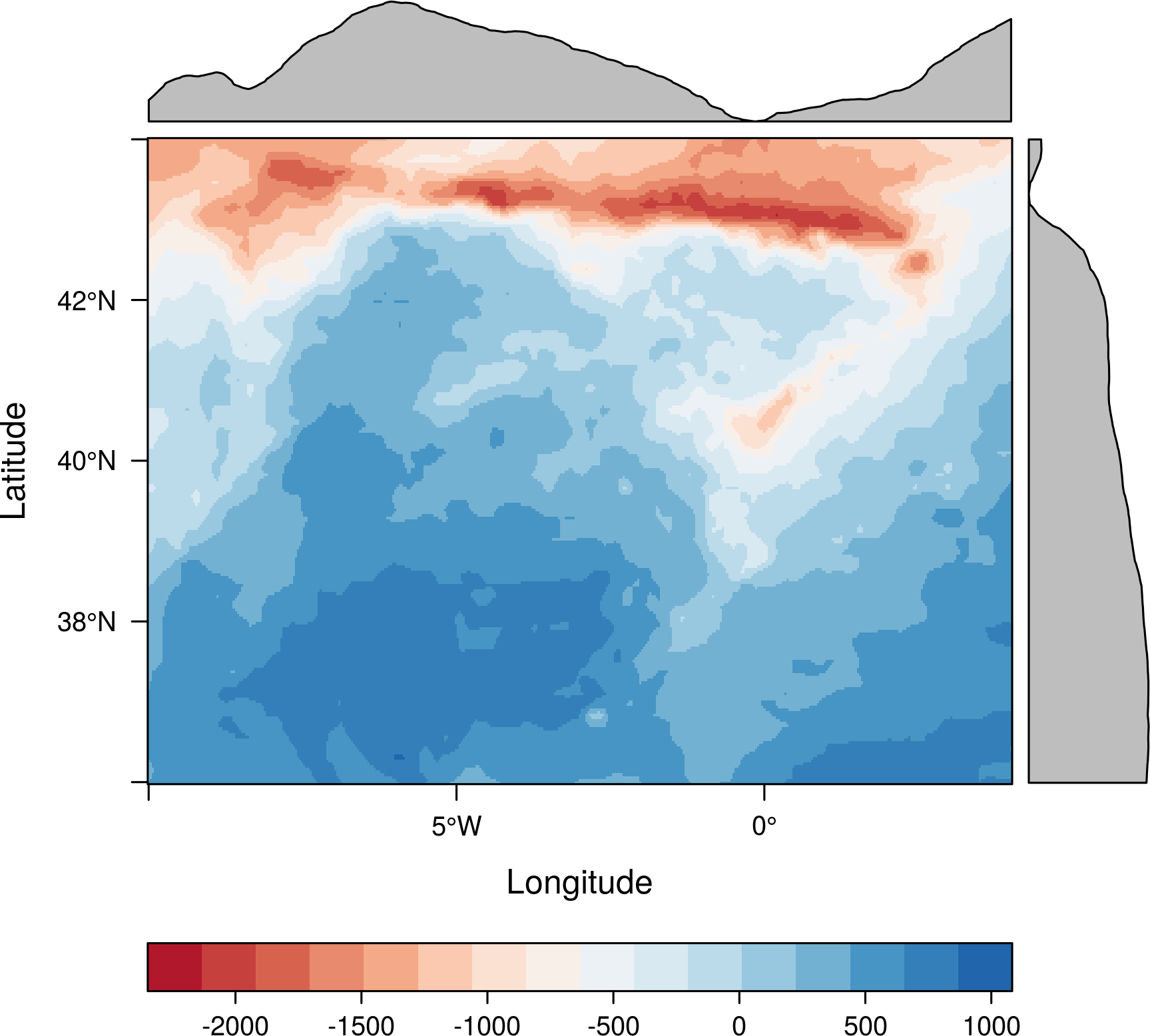Besides, it is easy to define a new theme with a different palette. For example, using a sequential palette from colorspace:

```library(colorspace)
myTheme <- rasterTheme(region=sequential_hcl(10, power=2.2))
levelplot(Aug, par.settings = myTheme, contour = TRUE)
```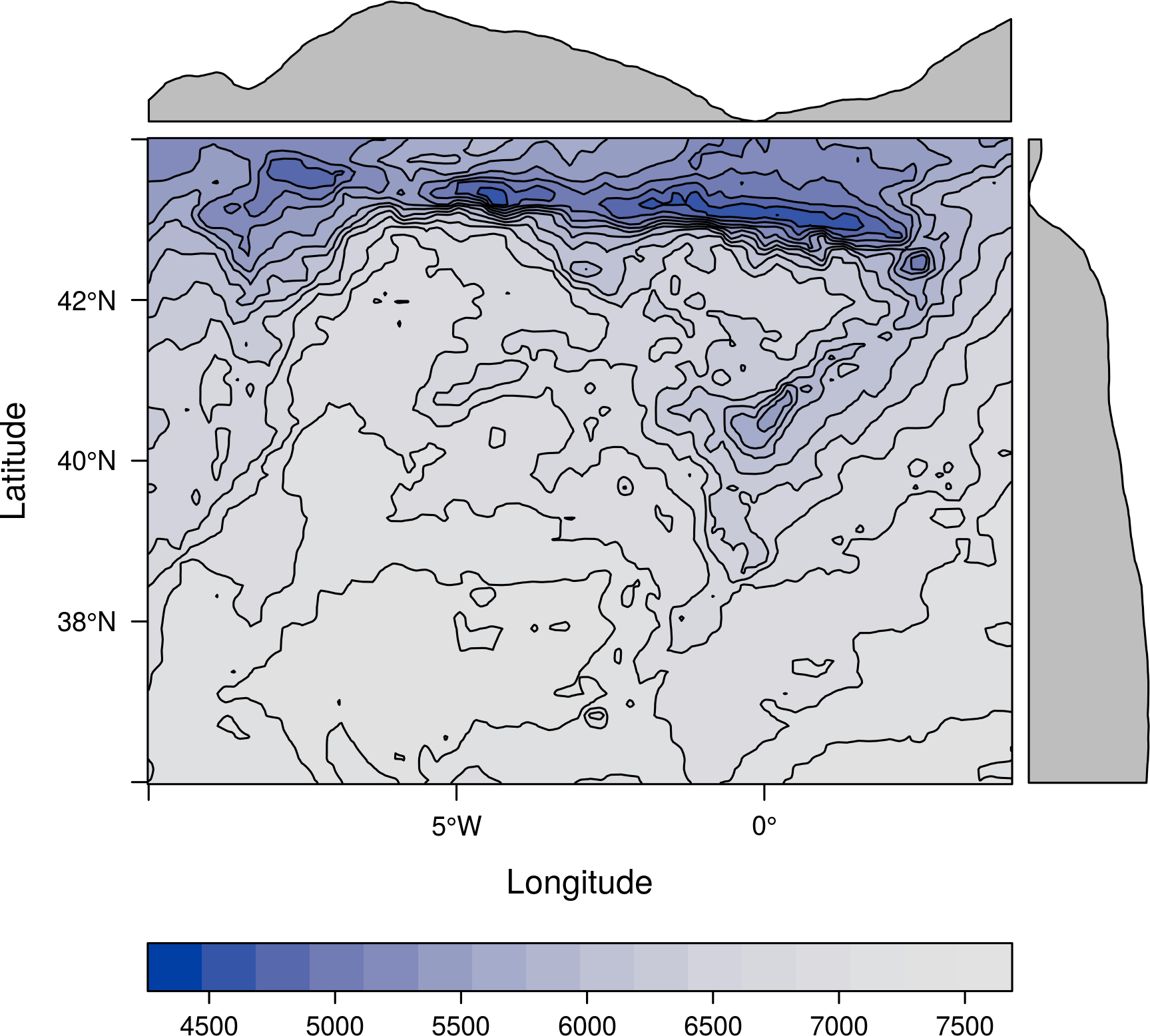or with the colour-blindness corrections from the dichromat package:

```library(dichromat)
myTheme <- rasterTheme(region = dichromat(terrain.colors(15)))
levelplot(Aug, par.settings = myTheme)
```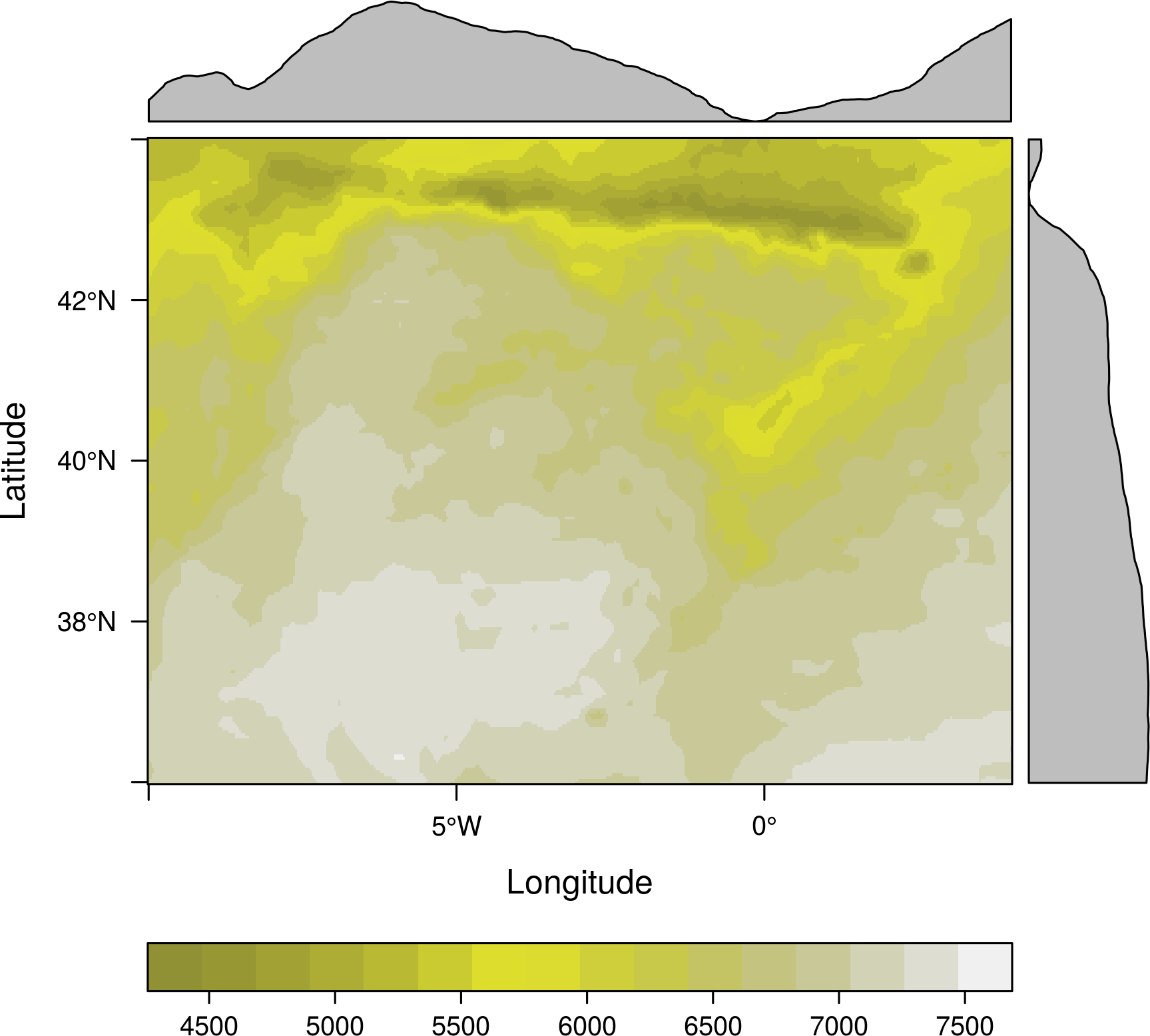## Categorical data

A raster that contains categorical data can be defined with the `ratify` function.

```r <- raster(nrow=10, ncol=10)
r[] = 1
r[51:100] = 3
r[3:6, 1:5] = 5
r <- ratify(r)
```

The levels are stored in the “Raster Attribute Table” (RAT) that can be manipulated with the `levels` function:

```rat <- levels(r)[]
rat\$class <- c('A1', 'B2', 'C3')
levels(r) <- rat
```

Such type of rasters are easily displayed with `levelplot`:

```levelplot(r, col.regions=c('palegreen', 'midnightblue', 'indianred1'))
```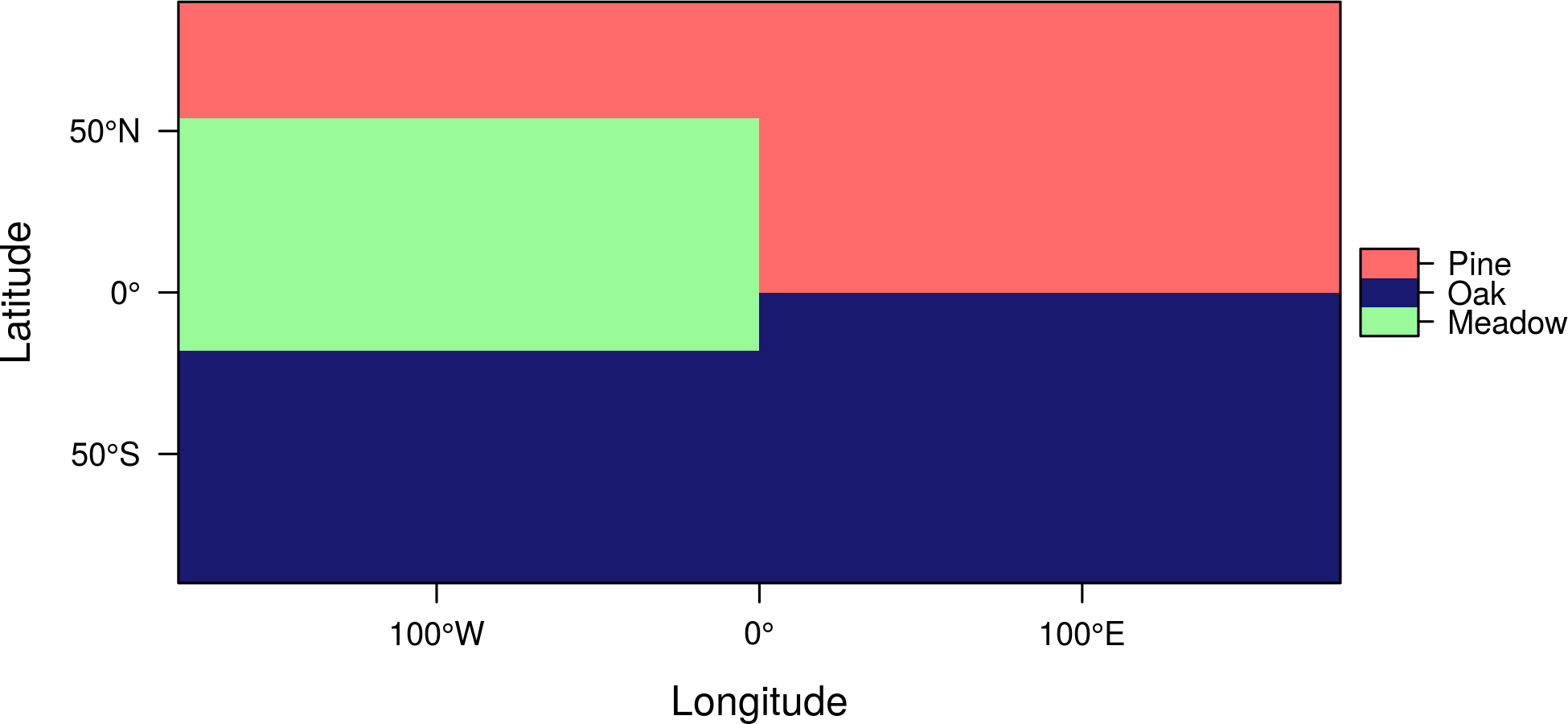You can choose the variable (column) from the RAT with the `att` argument:

```levelplot(r, att='class', col.regions=c('palegreen', 'midnightblue', 'indianred1'))
```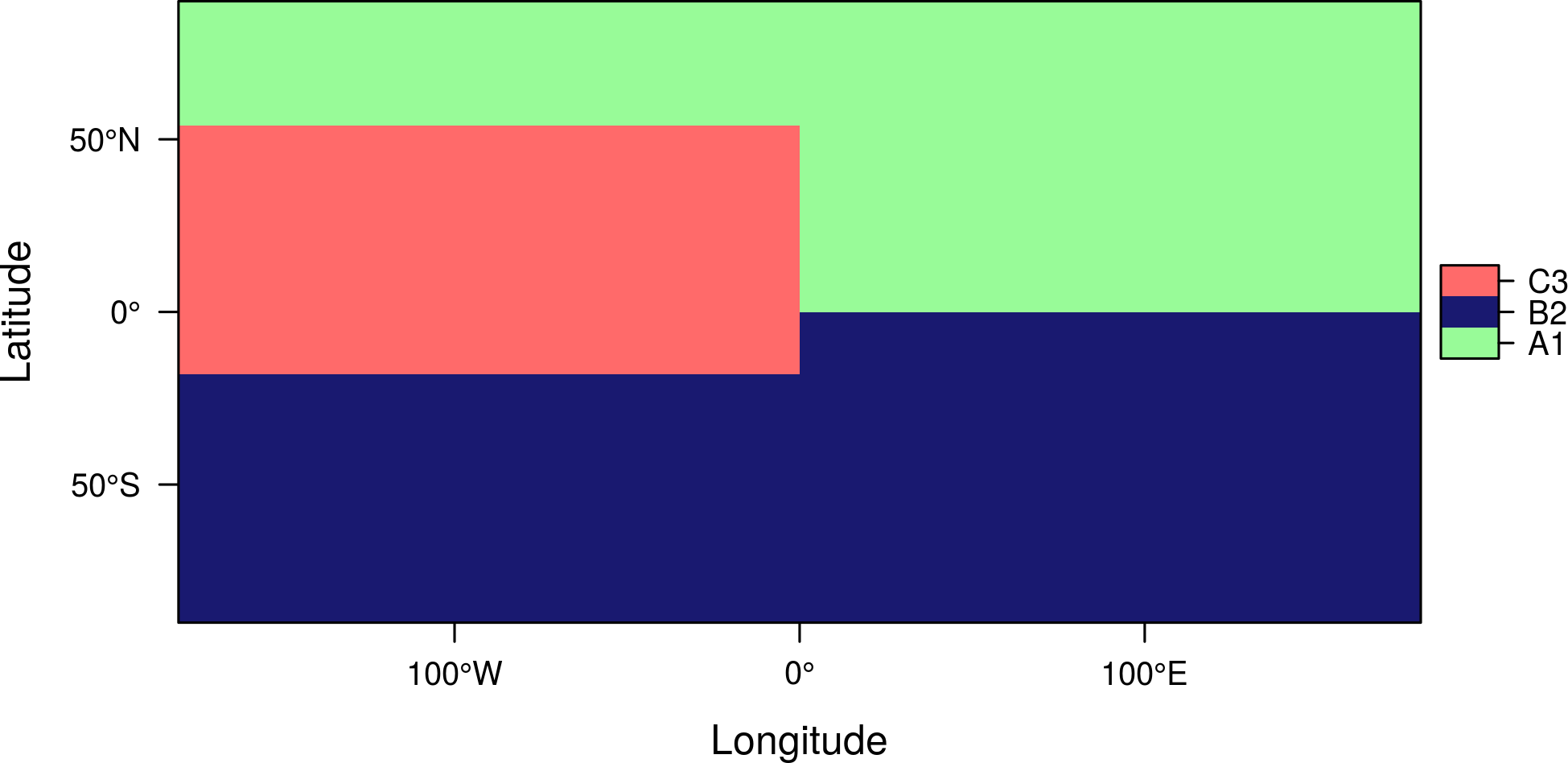## Scatterplots and histograms

There are methods to show scatter plots and hexbin plots of the layers and coordinates of a `Raster` object:

```  ##Relation between the January & February versus July radiation for four
##differents longitude regions.
xyplot(Jan+Feb~Jul|cut(x, 4), data = SISmm, auto.key = list(space='right'))
```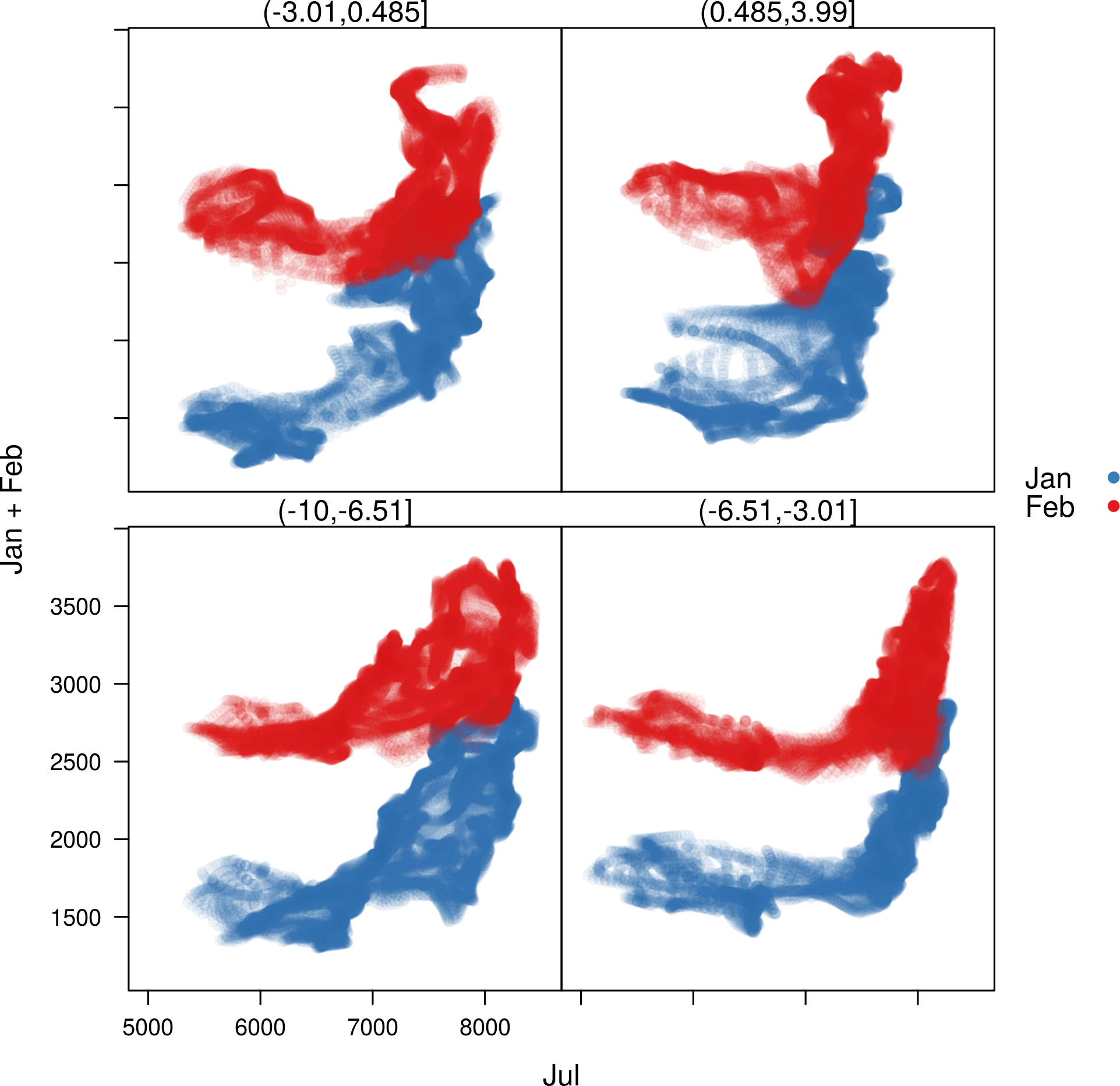```  ##Faster with hexbinplot
hexbinplot(Jan~Jul|cut(x, 6), data = SISmm)
```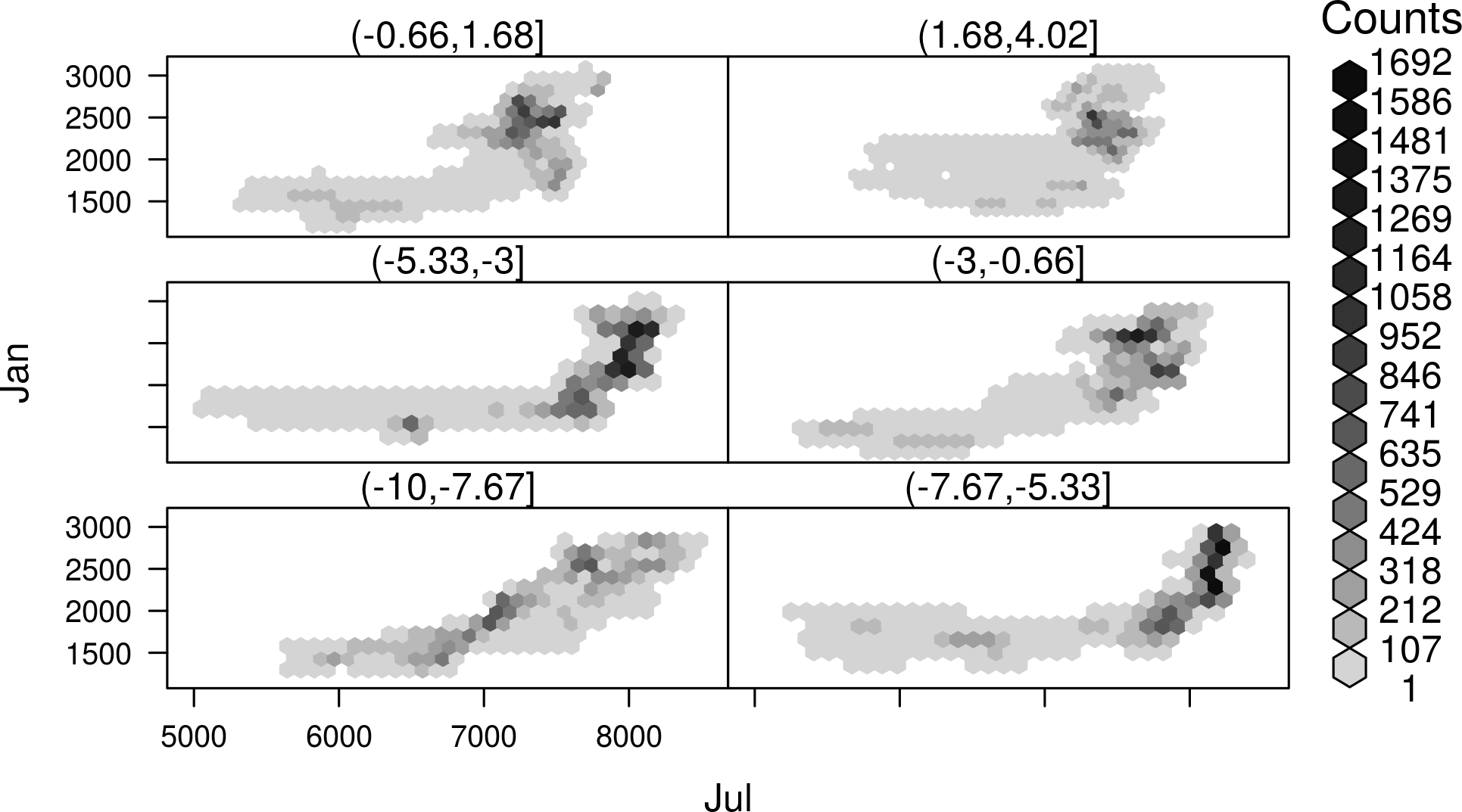…a method for scatter plot matrices:

```splom(SISmm)
```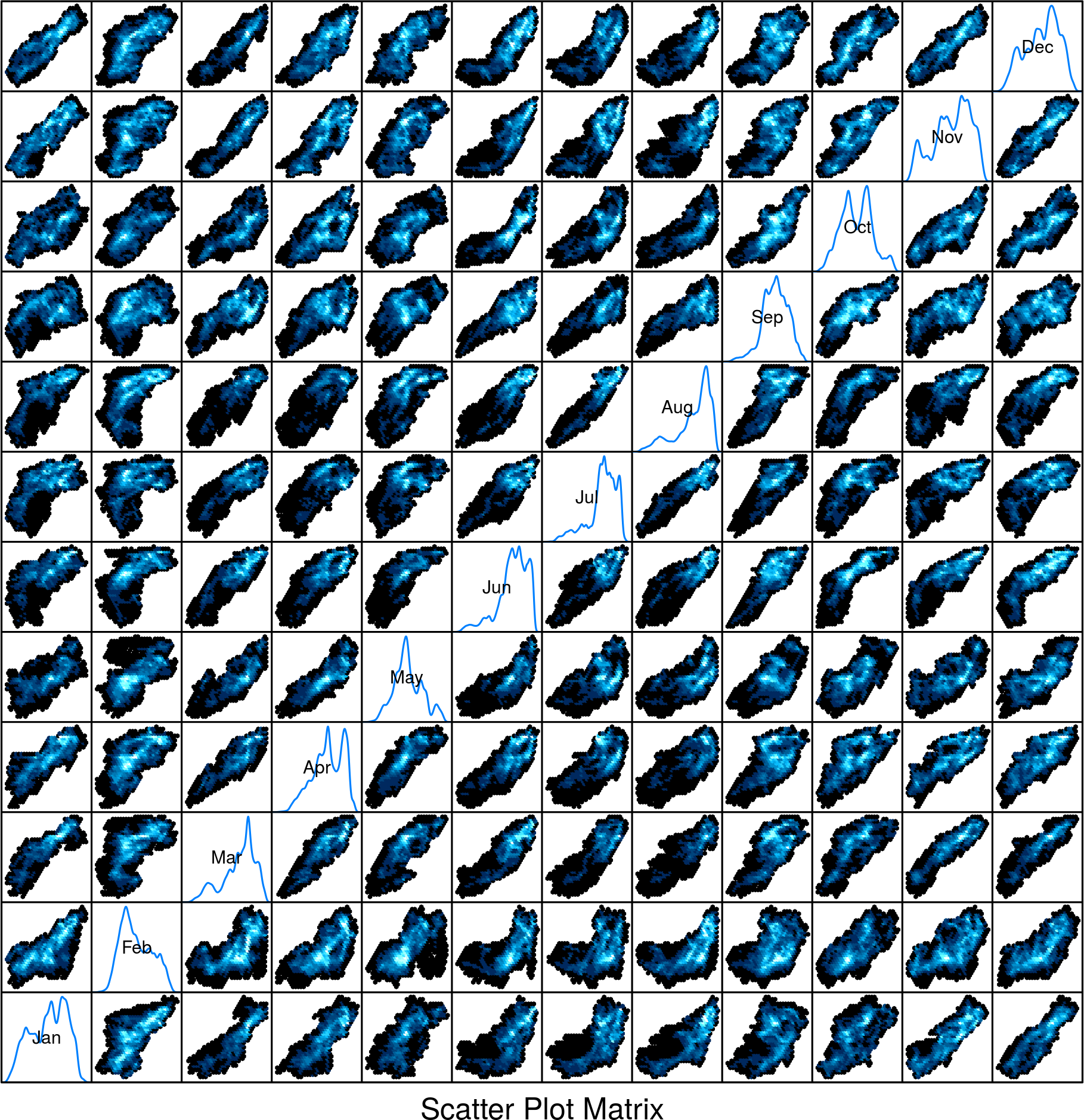..and methods for histograms, box-and-whisker and violin plots or density estimates:

```histogram(SISmm)
```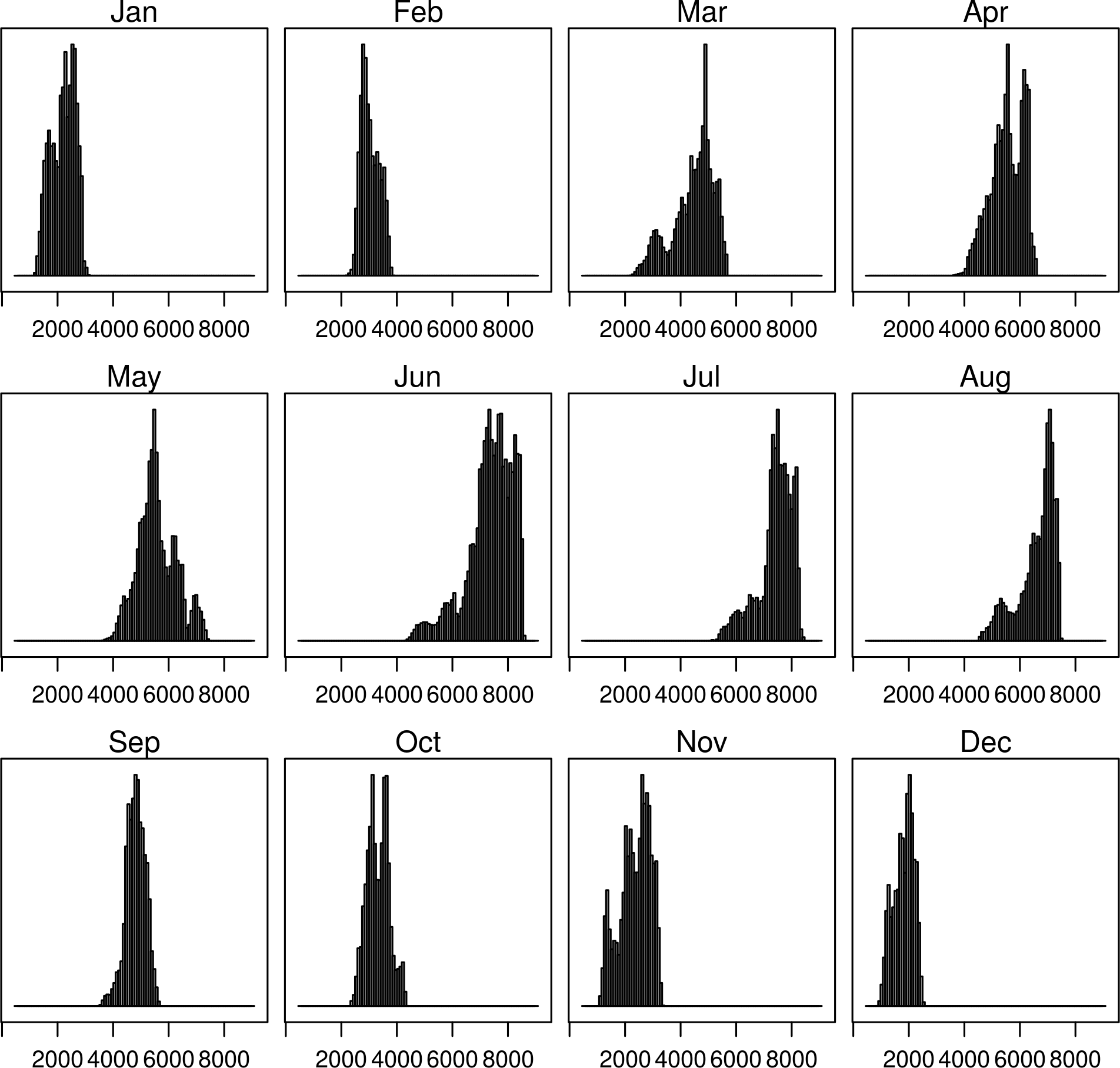```densityplot(SISmm)
```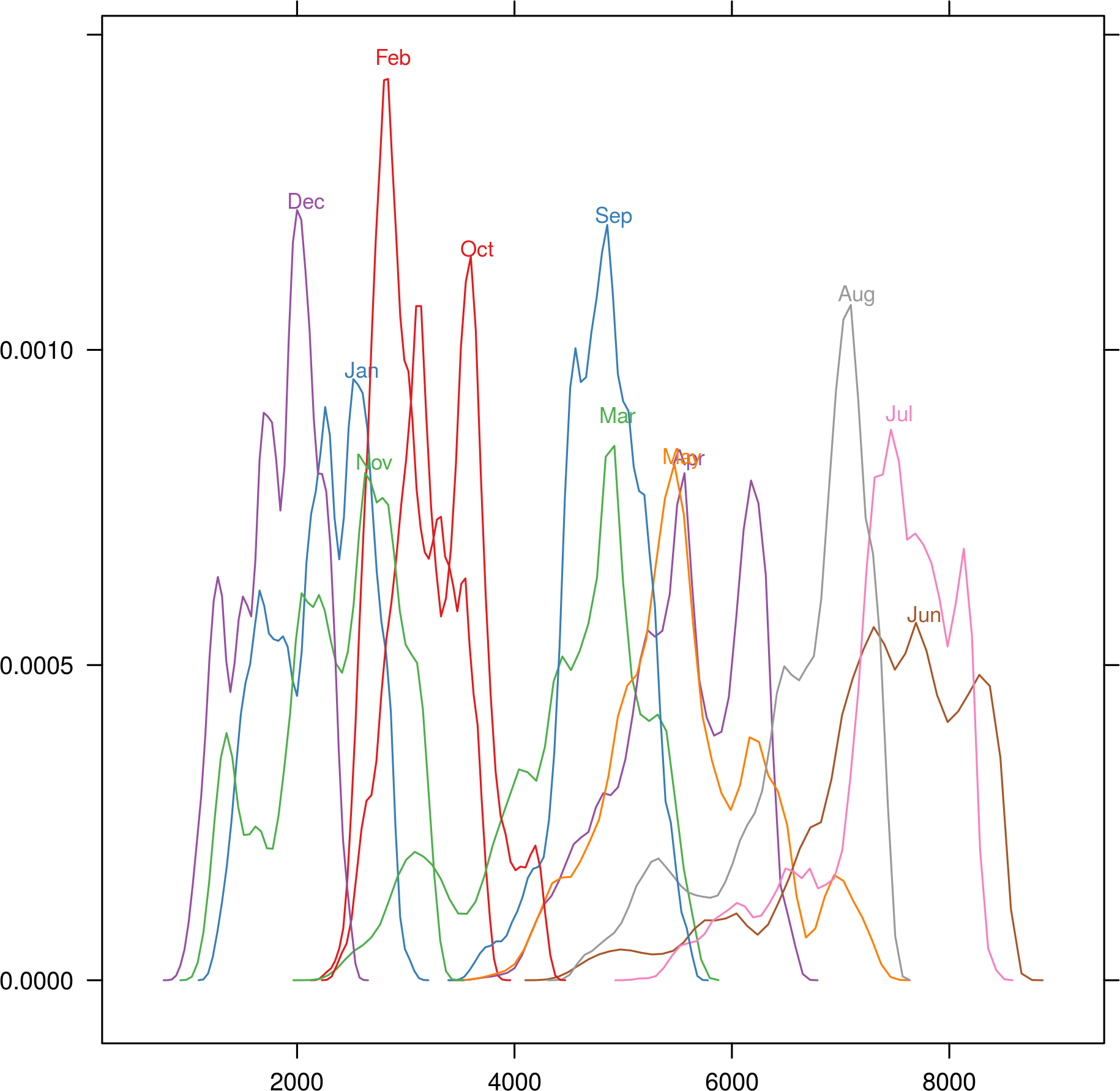```bwplot(SISmm)
```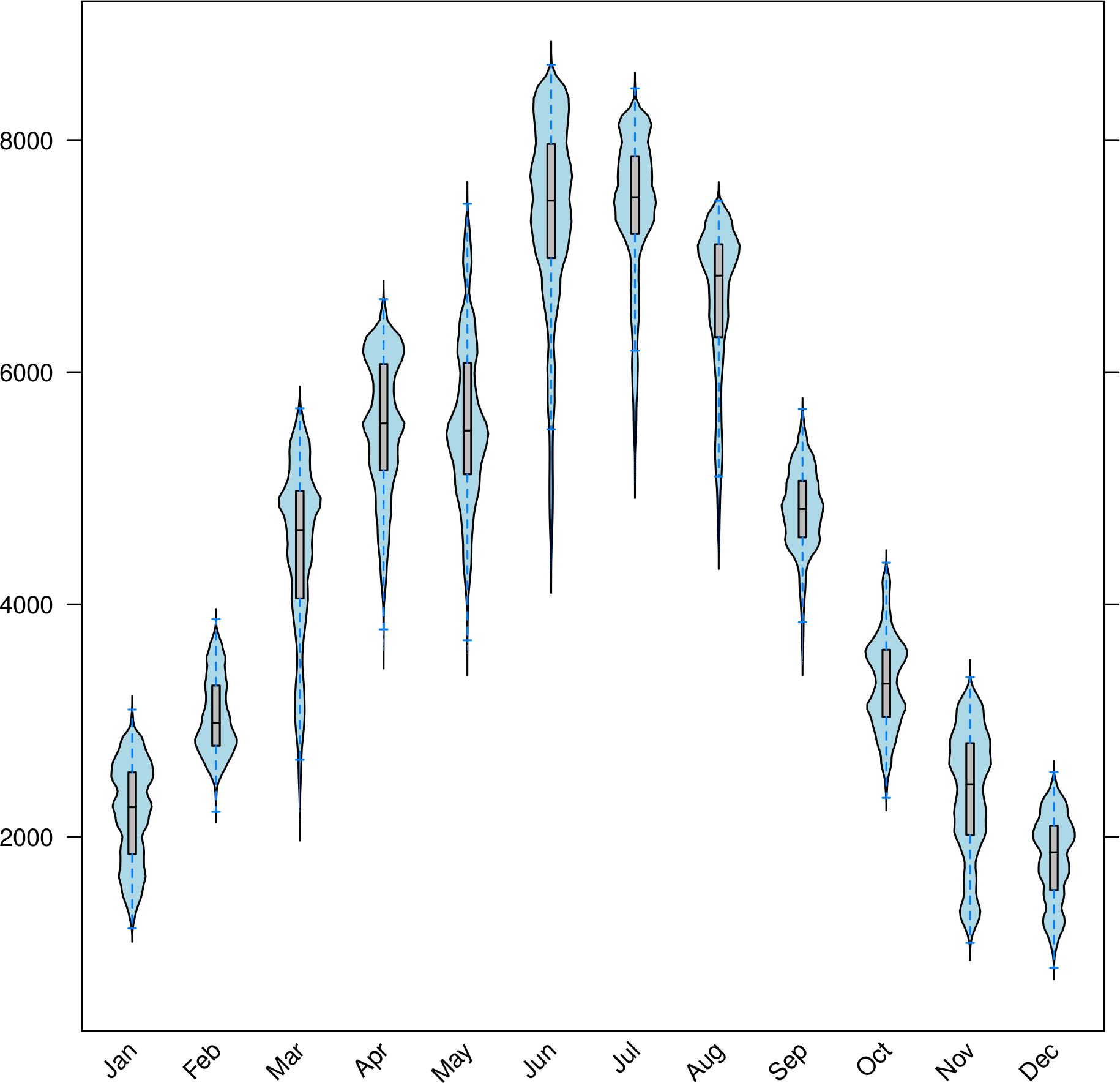These methods accept a `FUN` argument to be applied to the `z` slot of the `Raster` object. The result of this function is used as the grouping variable of the plot:

```histogram(SISmm, FUN = as.yearqtr)
```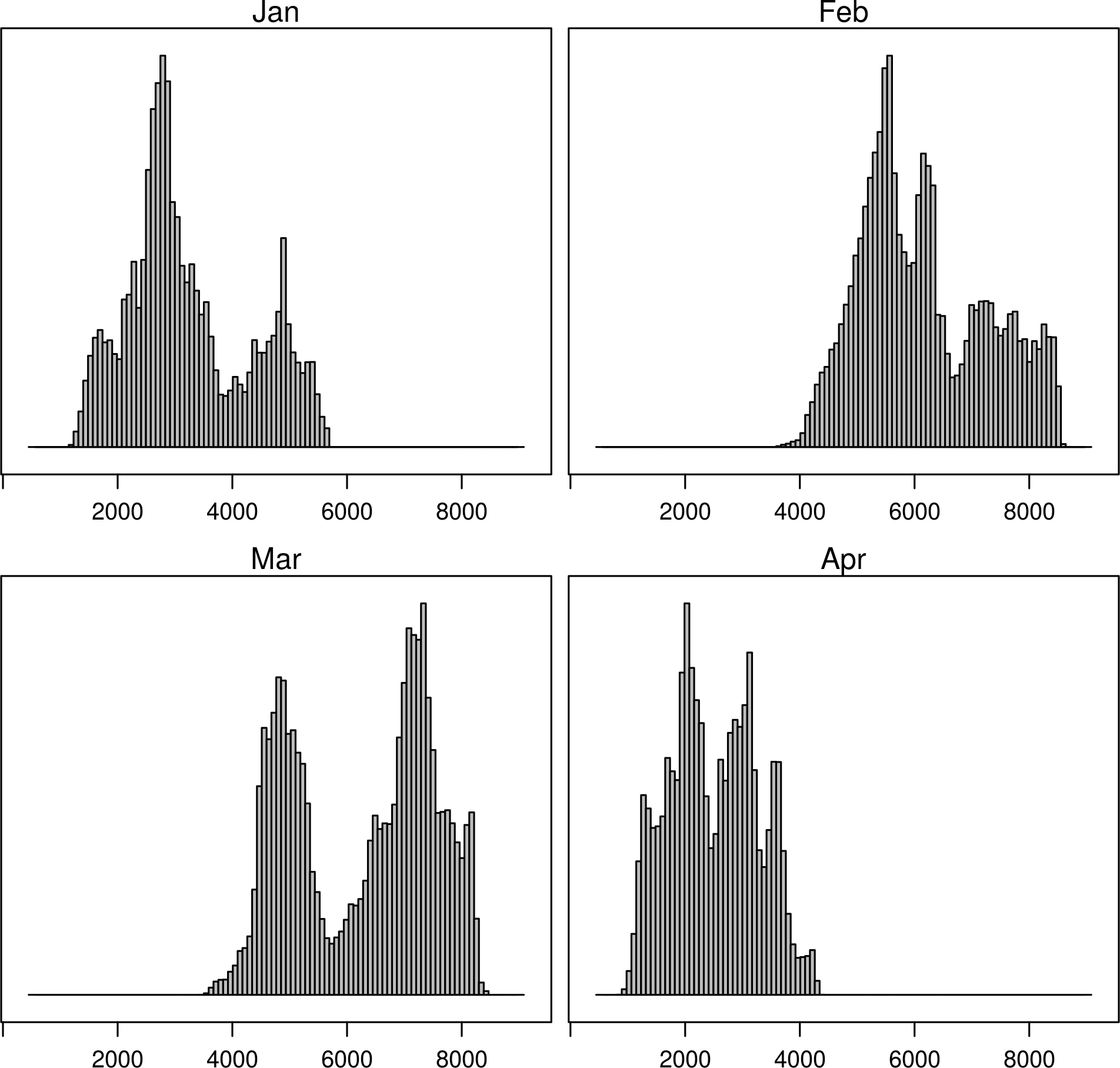## Space-time plots

The `z` slot of this `Raster` object stores a time index. This 3D space-time `Raster` object can be displayed with a hovmoller diagram.

The `hovmoller` method uses the function `xyLayer`, which creates a `RasterLayer` from a function of the coordinates.

```f <- system.file("external/test.grd", package = "raster")
r <- raster(f)
dirXY <- xyLayer(r, sqrt(x^2 + y^2), vector = FALSE)
dirXY
```

For example, the next code builds a hovmoller diagram showing the time evolution of the anomalies of Sea Surface Temperature data available from the Climate Analysis Center (more information here):

```library(zoo)

old <- setwd(tempdir())
destfile = 'SST.nc')
SST <- stack('SST.nc')
idx <- seq(as.Date('1970-01-01'), as.Date('2003-03-01'), by='month')
tt <- as.yearmon(idx)
SST <- setZ(SST, tt)
names(SST) <- as.character(tt)

## Extract month value from a Date or yearmon object
month <- function(x)format(x, '%m')
## Compute anomaly using monthly grouping with ave
anomaly <- function(x){
## Monthly means
mm <- ave(x, month(tt), FUN = mean)
## Monthly standard deviation
msd <- ave(x, month(tt), FUN = sd)
## anomaly
(x - mm)/msd
}

## Use anomaly with calc
SSTanom <- calc(SST, anomaly)
SSTanom <- setZ(SSTanom, tt)
setwd(old)
```
```## Ok, let's see the result
hovmoller(SSTanom,
at = seq(-3, 3, .25),
panel = panel.levelplot.raster,
interpolate = TRUE,
yscale.components = yscale.raster.subticks,
par.settings = BuRdTheme)
```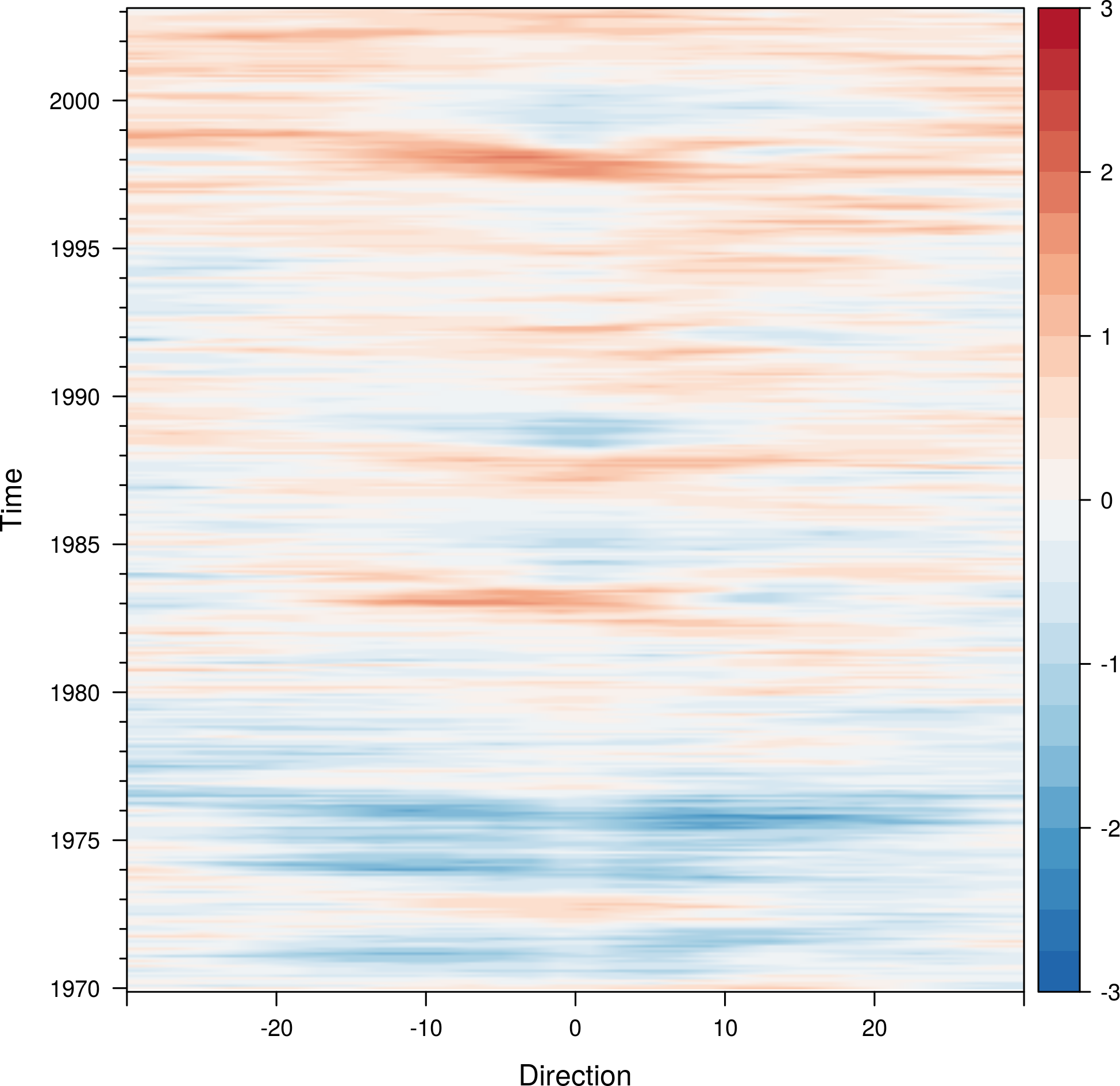The `horizonplot` and `xyplot` methods also are useful for the space-time `Raster` objects:

```horizonplot(SSTanom,
col.regions = rev(brewer.pal(n = 10, 'RdBu')))
```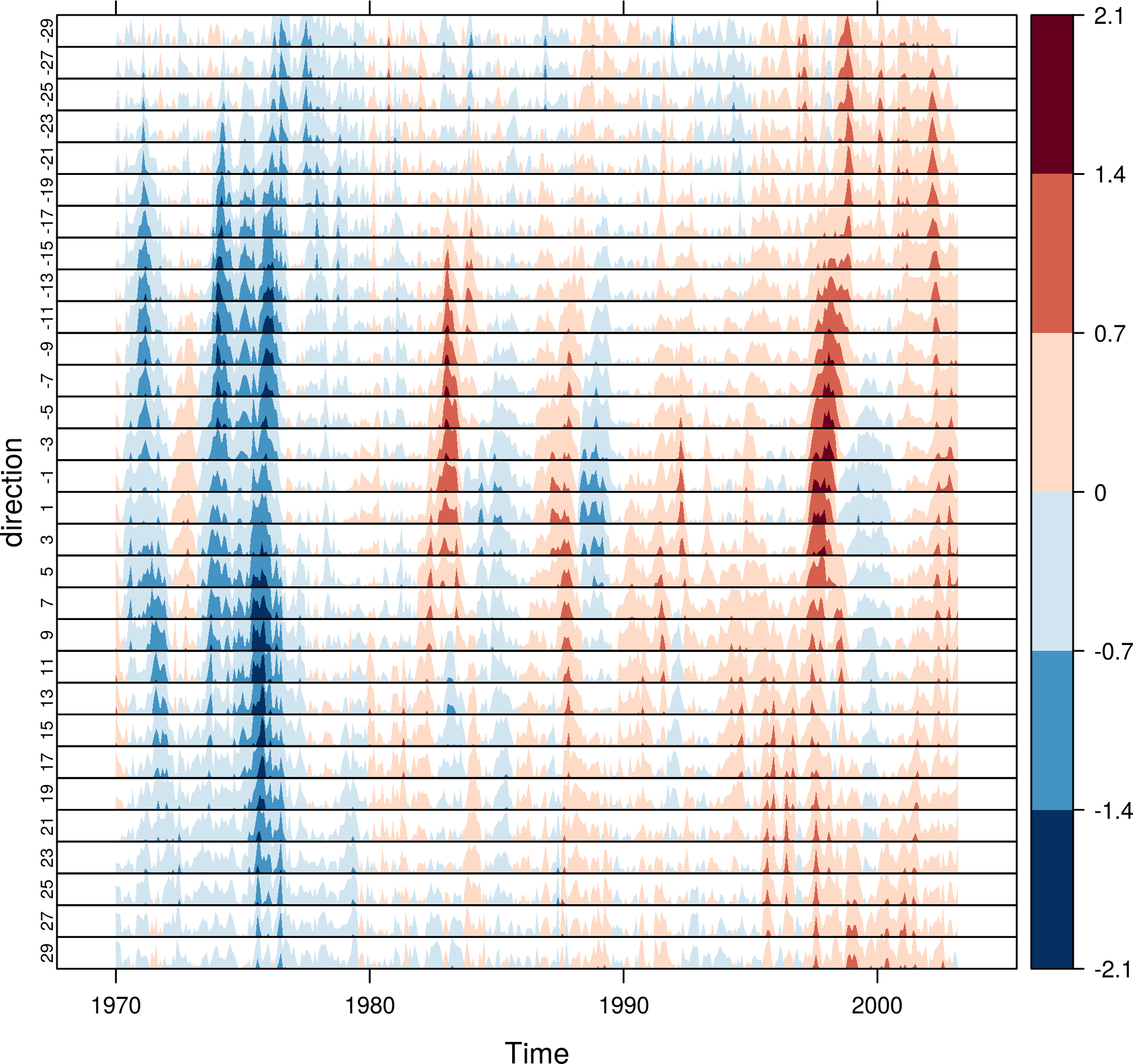## Vector field plots

The function `terrain` from `raster` provides the vector field (gradient) from a scalar field stored in a `RasterLayer` object. The magnitude (slope) and direction (aspect) of the vector field is usually displayed with a set of arrows (e.g. `quiver` in Matlab).

`rasterVis` includes a method, `vectorplot`, to calculate and display this vector field.

```proj <- CRS('+proj=longlat +datum=WGS84')
df <- expand.grid(x = seq(-2, 2, .01), y = seq(-2, 2, .01))

df\$z <- with(df, (3*x^2 + y)*exp(-x^2-y^2))
r <- rasterFromXYZ(df, crs=proj)
```
```vectorplot(r, par.settings=RdBuTheme())
```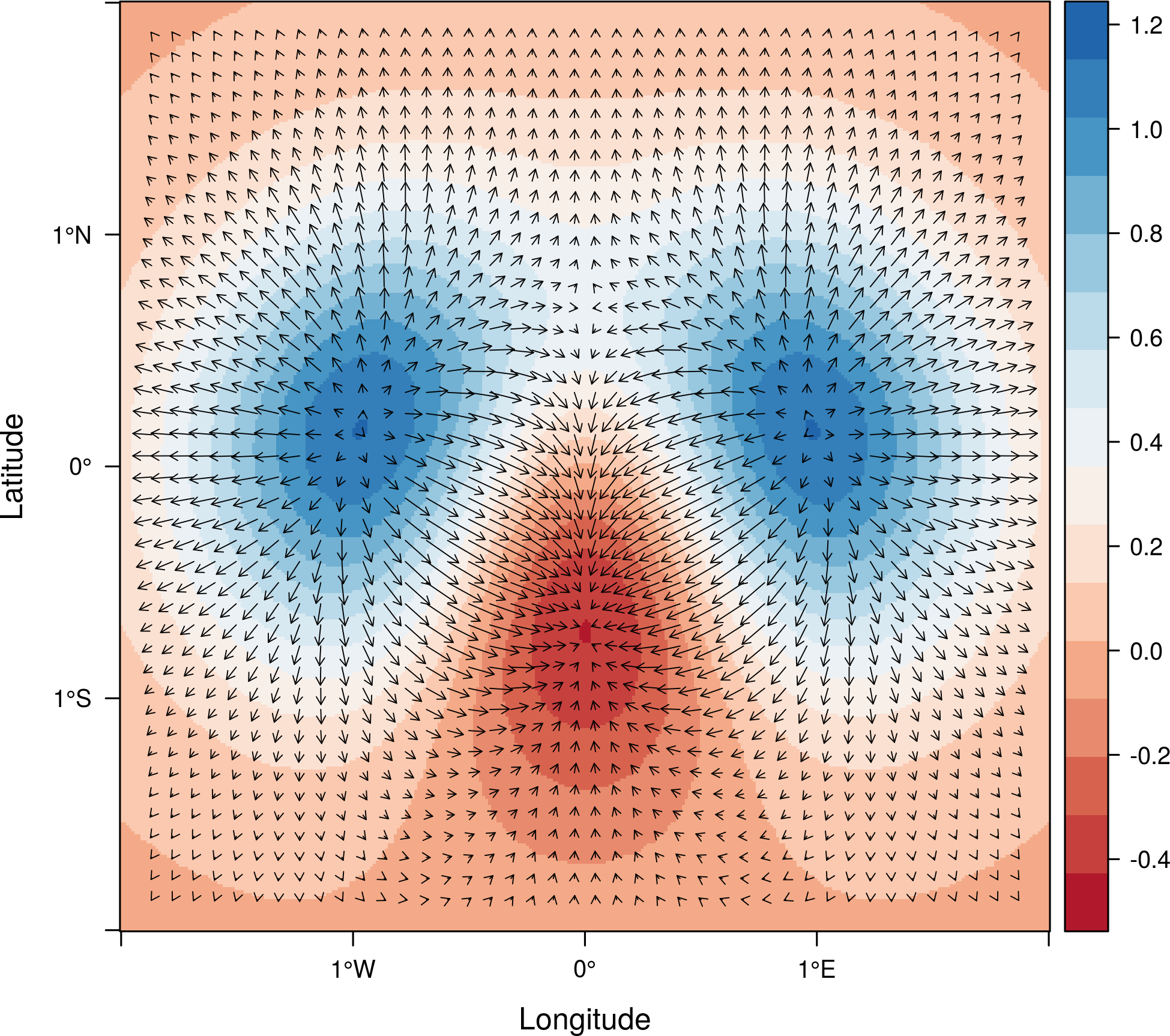If the `Raster*` object passed to `vectorplot` is a vector field (`isField=TRUE`), the `terrain` calculation is skipped.

An alternative method to display a vector field plots streamlines along the field lines. Streamlines, a family of curves that are tangent to the vector field, show the direction an element (droplet) will follow under the effect of the field. `streamplot` displays streamlines with a procedure inspired by the FROLIC algorithm: for each point (droplet) of a jittered regular grid, a short streamline portion (streamlet) is calculated by integrating the underlying vector field at that point. The main color of each streamlet indicates local vector magnitude (`slope`). Besides, streamlets are composed of points whose sizes, positions and color degradation encode the local vector direction (`aspect`).

```streamplot(r)
```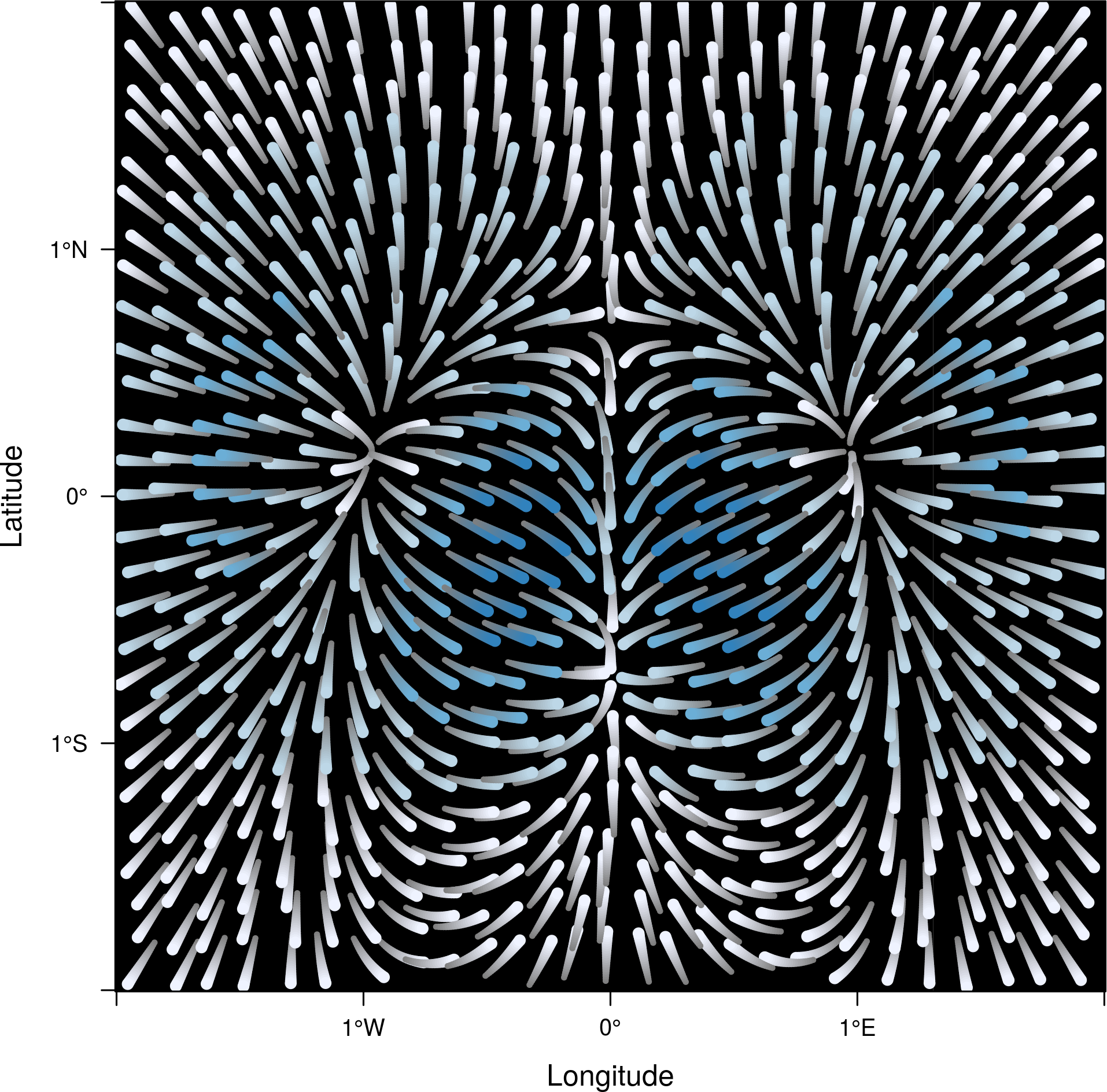`streamplot` accepts two arguments (`droplets` and `streamlets`) to control the number of droplets, the length of the streamlets and the streamlet calculation step. The streamlet colour palette and the panel background color are defined with an specific theme for `streamplot`, `streamTheme`. The default options can be changed easily:

```df\$z <- with(df, sqrt(x^2 + y^2))
df\$phi <- with(df, atan2(-y, x))
r2 <- rasterFromXYZ(df, crs=proj)

streamplot(r2, isField=TRUE, streamlet=list(L=30), droplet=list(pc=.3),
par.settings=streamTheme(symbol=brewer.pal(n=5, name='Reds')))

```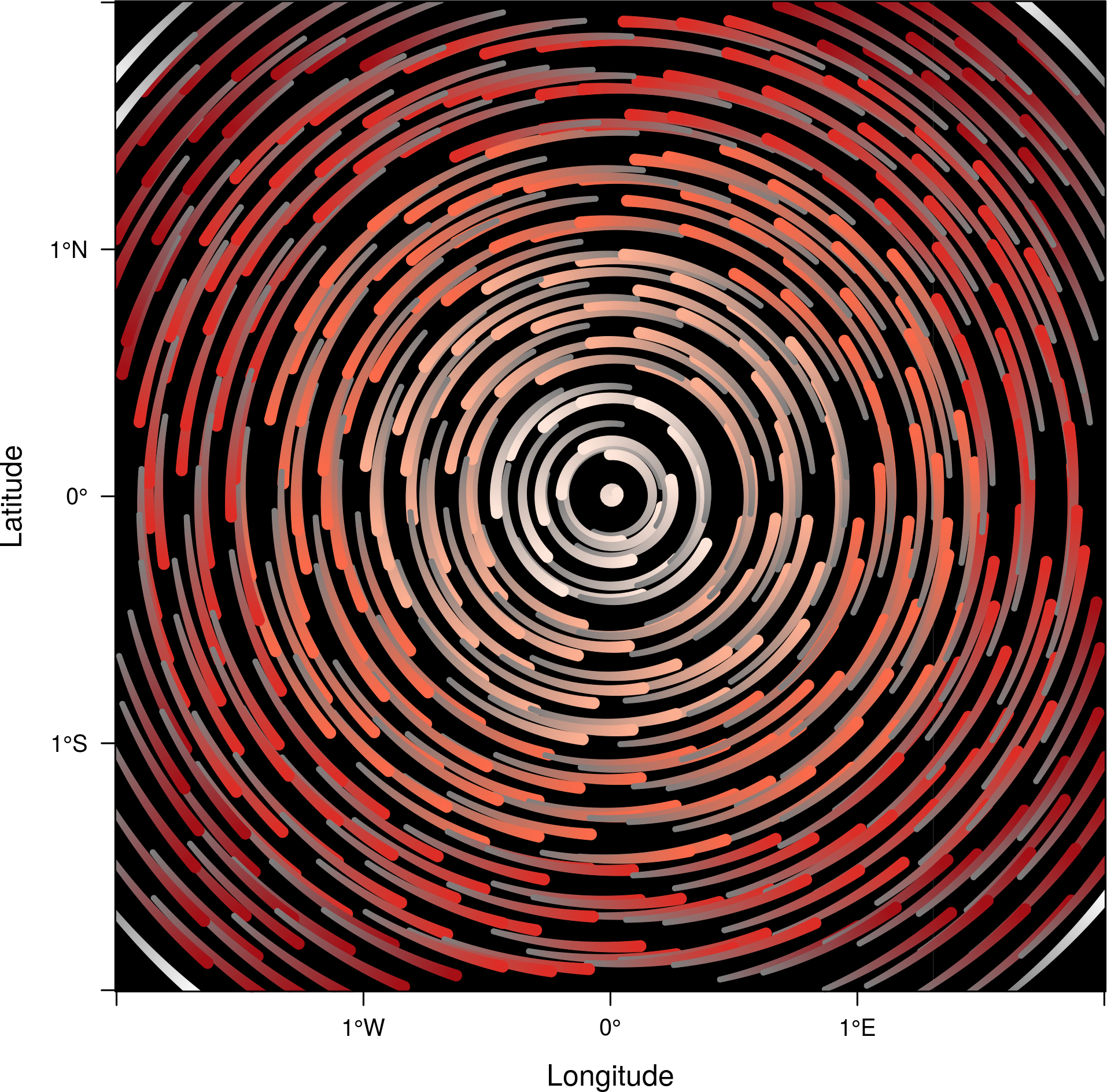## Interaction

This package includes two functions to interact with the `trellis` objects.

The `identifyRaster` method labels and returns points of a trellis graphic according to mouse clicks. It is commonly used after `levelplot`, although it can be also used after `xyplot`, `hexbinplot` or even `splom`:

```levelplot(SISmm)

## Do not close the last graphical window.  Use the left button of the
## mouse to identify points and the right button to finish

chosen <- identifyRaster(SISmm, layer=3, values=TRUE)
```

The `chooseRegion` function provides a set of points (in the form of a `SpatialPoints` object) inside a region defined by several mouse clicks. Use the left button of the mouse to build a border with points, and the right button to finish. The points enclosed by the border will be highlighted and returned as a SpatialPoints object.

```reg <- chooseRegion()
```

FAQs

View the Project on GitHub

Maintained by Oscar Perpiñán.